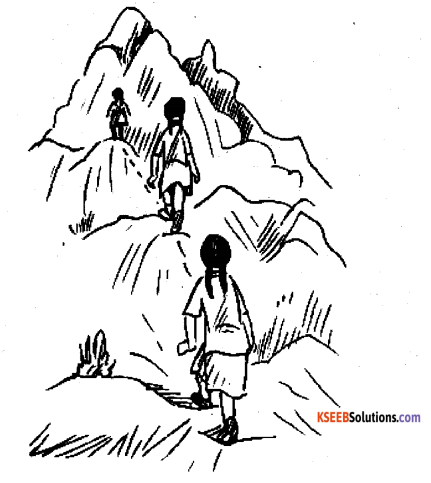# KSEEB Solutions for Class 6 Maths Chapter 11 Algebra Ex 11.4

Students can Download Chapter 11 Algebra Ex 11.4 Questions and Answers, Notes Pdf, KSEEB Solutions for Class 6 Maths helps you to revise the complete Karnataka State Board Syllabus and score more marks in your examinations.

## Karnataka State Syllabus Class 6 Maths Chapter 11 Algebra Ex 11.4

Question 1.
a) Take Sarita’s present age to be y years
Solution:
i) What will be her age 5 years from now?
Saritha’s present age + 5
= y + 5

ii) What was her age 3 years back
3 years ago, Saritha’s age = Saritha’s present age – 3
y – 3

iii) Sarita’s grandfather is 6 times her age. what is the age of her grandfather?
Grand father’s age = 6 × Sarita’s present age = 6y

iv) Grandmother is 2 years younger than grandfather. What is grandfather’s age?
Solution:
Grand father’s age = Grand father’s present age – 2 = 6y – 2

v) Saritha’s father’s age is 5 years more than 3 times Saritha’s age. What is her
father’s age?
Father ’s age = 5 + 3 x saritha’s persent age = 5 + 3yb) The length of a rectangular hall is 4 meters less than 3 times the breadth of the hall. What is the length, if the breadth is b meters?
Solution:
Length = 3 × Breadth – 4
l = (3b – 4) metres

c) A rectangular box has height h cm. its length is 5 times the height and breadth is 10 cm less than the length. Express he length and the breadth of the box in terms of the height.
Solution:
Length = 5 × Height
l = 5h cm
Breadth = 5 × Height – 10
b = (5h – 10)

d) Meena, Beena and Leena are climbing the steps to the hill top. Meena is at step s, Beena is 8 steps ahead and Leena 7 steps behind, of steps to the hill top is 10 less than 4 times what Meena has reached. Express the total number of steps usings.Solution:
She at which Beena is = (step at which Meen is) + 8
= S + 8
Step at which Leena is = (steps at which Meena is ) – 7
= S = 7
Total steps = 4 × (step at which meena is) – 7
= S – 7
Total steps = 4 × (steps at which Meena is ) – 10
= 4s – 10

e) A bus travels at v km per hour. It is going from Daspur to Beespur, After the bus travelled 5 hours, Beespur is still 20 km away. What is the distance from Daspur to Beespur? Express it using v.
Solution:
Speed = vkm/hr
Distance travelled in 5hrs = 5 × v = 5v km
Total distance between Daspur and Beespur = (5v + 20) km

Question 2.
Change the following statements using expressions into statements in ordinary language.
(For example, Give salim scores r runs in a cricket match, Nalin scores (r +15) runs. In ordinary language – Nalin scores 15 runs more than salim)
a) A note book costs Rs P.A book costs Rs 3 p.
Solution:
A book costs three times the cost of a note book

b) Tony puts q marbles on the table. He has 8q marbles in his box.
Solution:
Tony’s box contains 8 times the numbers of marbles on the tablec) Our class has n students. The school has 20n students.
Solution:
Total number of students in the school is 20 times that of our class.

d) Jaggu is Z years old. His uncle is 4z years old and his aunt is (4z – 3) years old.
Solution:
Jaggu’s uncle is 4 times older than jaggu and jaggu’s aunt is 3 years younger than his uncle.

e) In an arrangement of dots there are r rows. Each row contains 5 dots.
Solution:
The total number of close is 5 times the number of rows.

Question 3.
a) Given Munnurs age to be x years, can you guess what (9x – 2) may show?
(Hint: Think of Munnu’s younger brother.)
Can you guess what (x + 4) may shows? What (3x + 7) may show?
Solution:
(x – 2) represents that the person whose age is (x – 2) years, is 2 years younger to munnu
(X + 4) represents that the person, Whose age is (x + 4) Years, is 4 years elder to munnu
(3x+7) represents that the person whose age is (3x + 7) years, is elder to munnu and his age is 7 years more than three times of the age of munnu

b) Given sara’s age today to be y years. Think of her age in the future or in the past. What will the following expression indicate? y + 7, y – 3, y + 4$$\frac{1}{2}$$, y – 2$$\frac{1}{2}$$
Solution:
in future
After m years from now, sara’s age will be (y + h) years in past n years ago , sara’s age was (y – n) years
(y + 7) represents that the person, whose age is (y + 7) years, is 7 years elder to sara (y – 3) represents that the person, whose age is (y – 3) years, is 3 years younger to sara
(y + 41/2) represents that the person

Whose age is (y + 4$$\frac{1}{2}$$ ) years, is 4 1/2 years elder to sara (y – 2 1/2) represents that the person
Whose age is (y – 2 1/2) years, is 2 1 /2 years younger to sarac) Given n students in the class like football, What may 2n show? What may $$\frac{n}{2}$$ show?
(Hint: .Think of games other than football)
Solution:
2n may represent the number of students who like either football or some other game such as cricket Where as 11/2 represents the number of students who like Cricket, out of the total number of students who like football.